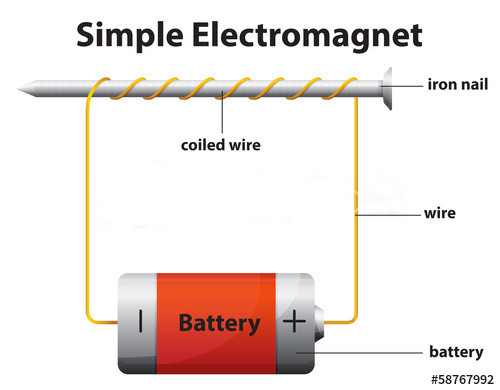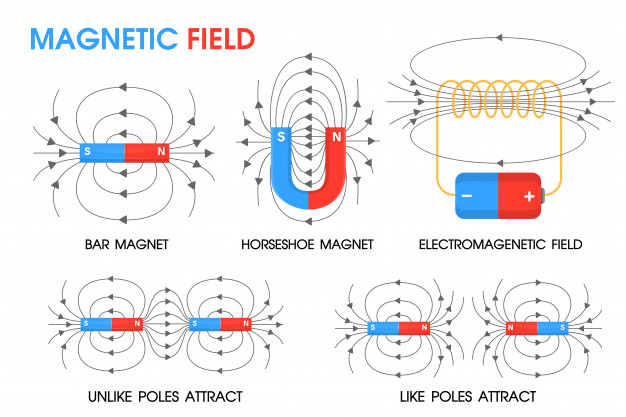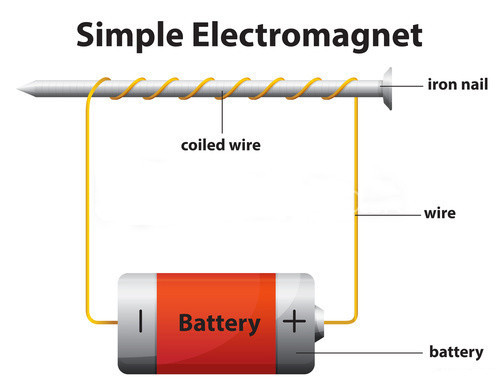# Lifting Power of Electromagnet – Ways to Increase Magnetic Field StrengthTo begin with, let’s familiarize ourselves with derived expressions for lifting power of an electromagnet: magnetic field, magnetic field strength.According to wikipedia, a magnetic field is a vector field that describes the magnetic influence on moving electric charges, electric currents, and magnetic materials. In electromagnetics, magnetic fields surround magnetized materials, and are created by electric currents such as those used in electromagnets, and by electric fields varying in time.

Lifting Power of Electromagnet (Magnetic Field Strength) Formula

Electric current(I) is the rate of flow of charge(Q) per unit time (t). i.e I=Q/t and Q=It from this equation, if you increase current, the quantity of charge also increases. In lorentz invariant equation of magnetic force:
F= qE+ qvB where
F is magnetic force
q is the quantity of charge
V is the velocity of the charged particles which are in our case electrons flowing in the conductors used to make the electromagnet, and B is the magnetic flux density.How Do You Make An Electromagnet Stronger?

This has the same meaning with questions like “how to make an electromagnet more powerful?”, “how can you increase the strength of an electromagnet?”, or “how to make an electromagnet stronger?”

From the above listed equations, we can clearly see how magnetic field strength depends on Current(I) and charge(Q). As the strength of the field from an electromagnet is controlled by and proportional to the current flowing through the coil and the number of turns – “ampere-turns” of the coil.

Therefore, there are several ways to increase electromagnetic strength:

Increasing the amount of current flowing through the wire – The magnetic field is caused by the current flowing in the wire. The bigger the current the stronger the magnetic field and hence the stronger the electromagnet.
However, it must be kept in mind that there is a limit to the strength of magnetic field the iron core will support. The current can be increased but the magnetic strength will not increase in proportion. The core saturates and an increase in current will not cause a corresponding increase in the magnetic field.

Adding a ferromagnetic core – this also increases the magnetic field (up to a point) and also helps to direct the field to where it’s needed. Using a large, continuous ferromagnetic core, combined with properly-shaped pole pieces, can concentrate the magnetic field and make it even stronger between pole pieces.
However, at high fields, ferromagnetic materials saturate, and provide no further benefit. The strongest electromagnets actually use no core – they are air-core coils. These typically operate with higher current and are usually water-cooled to remove heat. Even more powerful electromagnets use superconducting wire to prevent resistive losses.

Increasing the number of turns on core – Since the magnetic field induced by the electromagnet depends on number of turns of the coil per unit length it can be made more powerful by having more turns of wire in a given length of electro magnet.

Using material of high electric conductivity as coil (like copper, silver etc)

To sum up, the magnetic field strength of an electromagnet is proportional to both the number of turns in the winding, and the current in the wire. Increase in the magnetic strength means increasing the lifting power of an electromagnet.

### You May Also Like

© 2016 HVR Magnetics Co.,Ltd., is a professional manufacturer of electro permanent magnetic system.